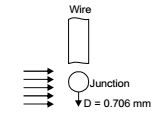## Heat Transfer Miscellaneous

#### Heat-Transfer

1. The average heat transfer coefficient on a thin hot vertical -plate suspended in still air can be determined from observations of the change in plate temperature with time as it cools. Assume the plate temperature to be uniform at any instant of time and radiation heat exchange with the surroundings negligible. The ambient temperature is 25°C, the plate has a total surface area of 0.1 m2 and a mass of 4 kg. The specific heat of the plate material is 2.5 kJ/kgK. The convective heat transfer coefficient in W/m2K, at the instant when the plate temperature is 225°C and the change in plate temperature with time dT/dt = – 0.02 K/s, is

1. From heat balance equation,

 -hA(T - T0) = mc dT dt

where, T0 = Ambient temperature
 ∴ h = 4 × 2.5 × 103 × 0.2 = 10 W /m2 K 0.1 × 200

##### Correct Option: A

From heat balance equation,

 -hA(T - T0) = mc dT dt

where, T0 = Ambient temperature
 ∴ h = 4 × 2.5 × 103 × 0.2 = 10 W /m2 K 0.1 × 200

1. A small copper ball of 5 mm diameter at 500 K is dropped into an oil bath whose temperature is 300 K. The thermal conductivity of copper is 400 W/mK, its density 9000 kg/m3 and its specific heat 385 J/kgK. If the heat transfer coefficient is 250 W/m2K and lumped analysis is assumed to be valid, the rate of fall of the temperature of the ball at the beginning of cooling will be, in K/s,

1. Given data: K = 400 W/mK; Ti = 500 K ; ρ = 9000 Kg/m3.
T = 300 K; Cp = 385 J/KG K; h = 250 W /m2K

 Lc = V = D = (0.005) = 8.33 × 10-4 A 6 6

 T = T∞ + (Ti - T∞) e -hAT ρcV

 dT = -hAT (Ti - T∞) e -hAT dt ρcV ρcVdT= hA (Ti - T∞) dt t = 0 ρcV

 = 250 × 200 = 17.31 K / S 9000 × 385 × 8.33 × 10-4

##### Correct Option: C

Given data: K = 400 W/mK; Ti = 500 K ; ρ = 9000 Kg/m3.
T = 300 K; Cp = 385 J/KG K; h = 250 W /m2K

 Lc = V = D = (0.005) = 8.33 × 10-4 A 6 6

 T = T∞ + (Ti - T∞) e -hAT ρcV

 dT = -hAT (Ti - T∞) e -hAT dt ρcV ρcVdT= hA (Ti - T∞) dt t = 0 ρcV

 = 250 × 200 = 17.31 K / S 9000 × 385 × 8.33 × 10-4

1. A spherical thermocouple junction of diameter 0.706 mm is to be used for the measurement of temperature of a gas stream. The convective heat transfer coefficient on bead surface is 400 W/m2K, Thermophysical properties of thermocouple material are k = 20 W/mK, c = 400 J/kgK and ρ = 8500 kg/m3. If the thermocouple initially at 30°C is placed in a hot stream of 300°C, the time taken by the bead to reach 298°C, is

1. Given h = 400 W/m2K, k = 20 W/mK
c = 400 J/kg.K, ρ = 8500 kg/m3 T(t) = 298 °C, Ti = 30 °C, T = 300 °C

 L = V = (π / 6)D3 = D A πD2 6

 = 1 × 0.706 × 10-3 = 1.76 × 10-4 m 6

 Biot Number = hL k

 = 400 × 1.176 × 10-4 = 0.0023 20

Since Bi < 0.1, lumped system analysis can be usedT(t) = e -hAT Ti - T∞ ρcv

 298 - 300 = e -hAT 30 - 300 ρcV

 -2 = e -hAT 270 ρcV

 ln270= hAT 2 ρcV

 ⇒ T = ρcV ln270= ρcVln 135 cA 2 h A

 = 8500 × 400 × 1.176 × 10-4 × 4.90 ≈ 4.9 sec. 400

##### Correct Option: B

Given h = 400 W/m2K, k = 20 W/mK
c = 400 J/kg.K, ρ = 8500 kg/m3 T(t) = 298 °C, Ti = 30 °C, T = 300 °C

 L = V = (π / 6)D3 = D A πD2 6

 = 1 × 0.706 × 10-3 = 1.76 × 10-4 m 6

 Biot Number = hL k

 = 400 × 1.176 × 10-4 = 0.0023 20

Since Bi < 0.1, lumped system analysis can be usedT(t) = e -hAT Ti - T∞ ρcv

 298 - 300 = e -hAT 30 - 300 ρcV

 -2 = e -hAT 270 ρcV

 ln270= hAT 2 ρcV

 ⇒ T = ρcV ln270= ρcVln 135 cA 2 h A

 = 8500 × 400 × 1.176 × 10-4 × 4.90 ≈ 4.9 sec. 400

1. The value of Biot number is very small (less than 0.01),when

1. When conductive resistance of solid is negligible, then biot number is very small.

##### Correct Option: C

When conductive resistance of solid is negligible, then biot number is very small.

1. Air enters a counter flow HE at 70°C and leaves at 40°C. Water enters at 30°C and leaves at 50°C, the LMTD in degree C is

1. ∴ ∆T1 = 70 – 50 = 20°C, ∆T2 = 40 – 30 = 10°C

 LMTD = ∆T1 ∆T2 log∆T1∆T2

 = 20 - 10 = 14.43 log2010

##### Correct Option: B

∴ ∆T1 = 70 – 50 = 20°C, ∆T2 = 40 – 30 = 10°C

 LMTD = ∆T1 ∆T2 log∆T1∆T2

 = 20 - 10 = 14.43 log2010